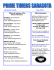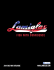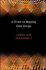# 17) Summa Brasiliensis Mathematicae, II

## Transcription

17) Summa Brasiliensis Mathematicae, II
```INSTITUTO BRASILEIRO DE EDUCACAO, CIENCIA E CULTURA
SUMMA
BRASILIENSIS
MATHEMATICAE
FASC 8
ON INTEGERS OF THE FORM 2k + p AND SOME
RELATED PROBLEMS
BY
P . ERDÖS
ANO V
NOVEMBRO DE 1950
VOL. II
ON INTEGERS OF THE FORM 2k+p AND SOME
RELATED PROBLEMS
By P . Erdös
Romanoff (1) proved that the integers of the form 2k +p, have
positive density . In other words, the- number of integers < x of
the form 2''+p is greater than c1x. Throughout this paper the c's
denote positive absolute constants . This result is surprising since
it follows-from the prime number theorem (or a more elementary
result of Tchebicheff) that the number of solutions in k and p of
2k + p < x is less than c2x . Romanoff (1) proved in fact the following result : Denote by f (n) the number r of solutions of 2k+p =n .
Then
1 X
(1)
lim .sup .
E f 2 (n) < o0
x n=1
The fact that the numbers of the form 2k+p have positive density follows immediately from (1), Schwartz's inequality and the
fact that the number of solutions in k and p of 2k+p < x is > c 3x.
Following a question of Turán (2 we first prove the following
THEOREM 1 . lim .sup .f (n) = co . Tn fact for infinitely many n
(2)
f (n) > c : log log n .
THEOREM
(3)
2.
For every k
lim . sup
E f k (n)
I x
. x
<
o0 .
nE1
Also following a question of Romanoff (3) we prove
THEOREM 3 .
There exists an arithmetic progression consisting
only of . odd numbers, no term of which i s of the form 2 1-'+ p.
Finally we prove
(4 ) Manuscrito recebido a 3 de Janeiro de 19 .50 .
(1) See e. g . Landau, Uber einige neuere Fortschritte der additiven Zahlentheorie . Cambridge
tract p. 63-70.
(2) Written communication .
(3) Written communication .
1 ' (SUM. BRAS . MATH . H --- False . 8, 113 - 1950)
P . ERDÖS
THEOREM 4 . Let a1 < a2 < . , be an infinite sequence of integers .
satisfying ak 1 ak+1 . Then the necessary and sufficient condition that
the sequence p -{-a k should have positive density is that
(4)
lim . supp .
log ak
Y
(5)
d; ai d
< 00
k
'
< C5 .
Finally we discuss a few related unsolved problems.
Proof of Theorem 1 .
cutive primes < (log
Define A as the product of the odd conseIt follows from the results of Tchebicheff (4 )
a,)/2 .
on -the function O(x) that
A < exp . O [(log x)''2 ] < exp 2 (log
x)'/2 ,
where exp z = ez .
Denote by S the number of solutions of
2k + p =- 0 (mod A), - 2k + p < x .
Let k _< log x, then if p < x/2, 2 k +
satisfying
p < x/2, . p -
p <
x . The number of primes
2k (mod A)
is, by the result of Rodosskii ( 5), greater than
[ 1 +0(1)1
x
2cp (A) log x
.
11+0(1)1
x
2 AA* log x
c xloglog x
A log x '
where
- II / 1 - 1 )
PlA \
1I
(1
log log x
(see
Thus by summing on k we obtain
(6)
S > cb x log log x/A .
(4) See e .g. the first few pages of Ingham's Cambridge tract on the distribution of primes or
Hardy and Wright's Number Theory .
5) On the distribution of prime numbers in short arithmetic progressions . Izvestiya Akad .
Nauk . S .S .S .R . Ser. Mat. 12, 123-128 (1948) .
(6) 4 ibid .
2 (Sum . BRAS . MATH . II - Faso . 8, 114 - 1950)
ON INTEGERS OF THE FORM 2k+p
But
z/A
1: f (kA) .
(7)
k=1
Hence from (6) and (7) we obtain that for some multiple 1A of A
x we have f (M) > c6 log log x, which proves Theorem
, the application .
not exceeding
If we only want to prove that lim sup J(n) (n) _
1.
of the prime number theorem f or arithmetic progressions would
have been sufficient .
It can be conjectured that J (n) = o(log n) .
This if true is probably
rather deep . I cannot even prove that for all sufficiently large n
not all the integers
n
(8)
can be prime.
k
1
:!r-
k .<
log n
log 2
For n - 105 all the integers (8) are prime, but it
is easy to see from a study of the prime tables that in the interval
105 < n
3 .52 .11 .13 .19=203775 there is no other such integer .
It seems likely that 105 is the largest exceptional integer . I believe
that the following result holds : Let c be any constant. and n t.T2<a1fsuhiec-rnxl_y,g>on.
exists- an
m so that the number of -solutions of m=-p+ai is
greater than c .
This would be a generalization of Theorem 1 .
Proof of Theorem 2 .
Denote by
pp,(x ; i1,
. . ., ik) the number
of solutions of the equations
pil + 2 1 _ pit '-L
(g)
in primes
r
pik + 2 1 k
A simple argument shows that
pti < x.
x
. y
212 - . . - -
fk(n) < kk[Y cp(x ; 1
. . . ,ik) +x
n=1
where" the summation is extended- over all the distinct i's satisfying
2i < x . (The first term of the right side of (10) comes from the n
with J (n)
3
> k, the second term from the n with J (n) < k) .
(Sum . BRAS. 'MATH. II
Fasc . 8, 115
1950)
P.
ERDÖS
Let g <X'7,- g prime c7 efficiently small, -and g does not divide
D, 1 : . s k =11 (2i u - 2i v), 1 << V < U < k . Then if p i l > xt'7 satisfies
(9), we clearly must have
Pi - • -- . (2i1
2' ,)
(mod - g) . false for r = 1, 2, .
. .,
k.
(since pi i +2= ,. 24, pi, is a prime) . Since g/Di 1 . . ik is false these
k residues are. all different . Thus ' by Brun's method - C?)
cp(x;
.,
i 1,
i k) <
xc7
1
+ C8 X IT 1
x`k ~
C9
\(log
2 ' 1 'F' g '
~
J
where . 11 1 -denotes the product for all g < x~7, g /
and I1 2 the product -for all g / D\$ 1 . . .ik .
Dti1 . . . ik
I
false
Now by the inequality of the geometric and arithmetic means
k (k ----1)/2
2
g
5
k (k 1)
2;
1 H, 3
9
where 2; 1 denotes the sum subject to the conditions 1 < V < U :5 k
and I13 the product for all g / T u - 2iv .
Thus by a simple argument
k (k -- I)/2
(12) 22 112
1
+
< C
9
1O (log x)k-2 Y II }
1+
+~
1
9
- on the left side of *(12) the summation Z2 being- extended over the
distinct sets of i's satisfying .2i < x and on the- right side Z3 denoting the sum subject to
log x
1 <v < 21
< l0g 2
and 11 4 the product- over all g / 2L 2 . Now trivially for a fixed
B=B (k)
k (k - 1)/2
B
(13)
1
< 1 +
9
9
(7) See e.g. P . 'Erdös, Proc . Cambridge Phil . Soc 33, 6-12 (1937) .
4 (SUM . BRAS . MATH. II -- Fast. 8, 116 . - 1959)
I
ON INTEGERS OF THE FORM
2'"+p
Thus to prove Theorem 2 it will suffice to show that (by (10), (11),
(12) and (13))
81
4 T14 (i+
< C 11 log x .
91
where Z4 denotes the sum subject to 2u <_ x and I1 4 refers to- the
product extended over all g / 2u -1 .
Now if l2 (d) denotes the smallest exponent z for which 2±-1 (mod d)
and v(d) denotes the number - of distinct prime factors of . d, then
b y a simple argument (interchanging the order of summation)
CO
Bl (d)
X4 114 1 +
<
9
;4 ~ ;
. d
< C12
Bn(d)
log x
d=1
d
12
(d)
where 1 5 refers to the sum subject 'to -d / 2" 1 .
Thus to complete the proof of Theorem 2 we have to prove that .
B (d)
v
(14)
~
d =1
d
12
(d)
The proof - of (14) will be very similar to the proof - ,given by Turin
-1
and myself (8) for the theorem of Romanoff
d=1
d
.2
< .
(d)
To prove (14) we split the integers into two classes . -In the first
class are the integers with 12(d) < (log d)'13, , c13 > B and in the
second class are the other integers . Clearly the .-integers . of the
first class. are all composed of the prime factors of the set(15)
2k
1
1 < k < (log
x)'13 . .
Since the number of prime factors of 2k -1 is clearly < k, the number
of the prime factors of the . sequence (15) is clearly < (log x) 2c13
Denote the prime factors of the sequence (15) by pl, p2,r,
r < (log x)2c13 . Clearly- the number of integers of the first , class is
less than the . number of -integers, < x composed of p l-, p2,
p
:entirely
of
the
p's
into
two
We split the integers < x composed
groups . In the first group are the integers having less than
D
log x / 4 , C 13 . log log x
(8) Ibid 1 p . 68 .
(Sum . BS"
. MATH. II -- Fasc . 8, 117 - 1950)
P. ERDÖS
different prime factors and in the second group are the other
integers compose d of the p's . The number of integers of the first
group is clearly less than
(16)
. . .-I- ( 0 )] X
C \DI + (Dr 1! +
`
log x
x
(lot x \
log 2
D
D
I
log 2
<
for x > x0 -
The factor (log x / log 2)" comes from the fact that the number of
log x
log X pS S x equals
log 2
log 2
The number of integers of the second group is clearly less than
r
(17)
x
Ei
D (i=
D
E
p~S
S
x
(c i t log log -log x) °< x 1-- 8
D
since D! > xa and
r
Ei=1 F,s
p y8
< c 15 log log r < c 14 log log log x .
Thus from (16) and (17) we obtain that the number of integers < x of
the first class is less than 2x 1-' for sufficiently large x. Denote by
d1 <d2
<
. . .- the
integers of the first class . We evidently have
for sufficiently large k
(18)
dk > x1 + s!2
Since v(d) < c 16 log d / log log d, we obtain by (18)
B v(d)
d lz (d)
I
< C 1 ; EI k 1 -{- 6/4 < o~
k=1
f
where in the left side of (19) the summation is . extended over the
integers of the first class, i . e . the integers satisfying 1 2 (d). :!-< (log d)'13 .
N ow we have to show that
(20)
By (d)
~dl2.
< CO
(d)
6 (SUM . BRAS . MATH. H -- Fasc . 8, 11 .8 - 1950)
ON INTEGERS OF THE FORM
2k+pF
wherein .(20) the summation is extended over the integers satisfying
In fact we shall prove that
12(d) > (log d)e=3 .
°°
.(
B
.
d _ 1, d (log d)' I3
and this clearly implies (20) .
By partial summation we . obtain
that to prove (21) it will suffice to show that
x
oa
6x
(22)
X =.: 1
x
2
log x)
where
cI3
or,
= d=1
E - Ba(d)
We 'have
x
x
xl
.2-(d) _
d=1
l=1
µ(i)0
= 0 z log x)
Assume now that
x
E
(B -- 1)v(d) . - 0 [x (log .x) B-2 ] .
dal
We then have by induction, interchanging the order of summation
and partial summation
x
~x =
d=1
(B 7 1) + 1]v(d) _
x
B
<
l
l=1
µ WOO
< x
1:x (B -I =1
l
x
1)v(l)
1 (log l) 8-- 2= 0 x
c x
l =1
l
2
[
(log x) B - 1 1 .
Thus (22) converges for cla > B . Hence from (1g) and (20) it follows
that (14) converges, which completes the proof of Theorem - 2 .
Proof of Theorem 3.
Clearly every integer, satisfies at least one
of the following congruences : 0 (mod 2), 0 (mod 3), 1 (mod 4), 3
(mod 8), 7 (mod 12), .23 (mod 24) . Therefore,. if x is congruent to :
I (mod 2), 1 (mod 7), 2 (mod 5), 2 3 (mod 17), '27 (mod 13), 223
(mod 241), then for any k, x - 2k is a multiple of one of, the primes
3, 5, 7, 13, 17,'241 . This proves Theorem 3 .
7 (Sum . BRAS . MATH. II -- Fast. 8, 119 - 1950)
P. ERDÖS
The reason . for the success of this proof is that ., by a well known
theorem (9), for any n;& 6 there exists a prime p satisfying p / 2' 1
and p /2" -1 false for all m < n .
The simplest system of congruences a i(mod-n ), n 1 < n2 < . . . <
n k so that every integer satisfies at least one of them is : 0 (mod 2),
0 (mod 3), 1 (mod 4),, 5 (mod 6), 7 (mod 12) . But because of the
modulus 6 this system cannot be used- :to prove Theorem 3.
The following such system does not contain . the modulus 2 :
0 (mod 3), 0 (mod 4), 0 (mod :5), 1 (mod 6), 6 (mod 8), 3 (mod 10),
5 (mod 12), 11 (mod 15), 7 - (mod 20), 10 (mod 24), 2 (mod 30),
34 (mod 40), 59 (mod 60), 98 (mod 120) . Davenport found a slightly
more complicated system somewhat earlier . it. seems likely that for
every c there exists such a system all the moduli of which are > c .
This - would imply, by the same argument which proved Theorem
3, that for every c' there exists an arithmetic progression no term
of which is of the form 2k + u, where v (u) < c' .
Proof of Theorem 4 .- The proof of the .necessity is easy. If (4)
is not satisfied then for- a suitable sequence n i the number "'of -the
ak -< n i -is o (log n) ±and 'since i ( n) < 2n~ / log n i we obtain that
the number of the integers of the form p±ak < n i is o (n), or (4)
is necessary .
To show the necessity of (5), - assume that (5) does not hold .
Let A be large and j chosen so large that E 1/d > A . Let n be
s
_sufficiently large . We. split the integers of the form sp + ak n
into three classes . In the first class are the integers 5 n of the form
p+ak,
k <j
the number of these integers is less than j 7r (n) - (n) .
The integers . of the second class satisfy p /'aj. The number of
these integers is clearly less than v(a;) times the number of the
a's not exceeding n, thus it is 0(log n) = o(n) .
In the third class are all the other integers of the form p + a k fl .
Clearly from a; / ak- for j < k and (p ;a;) 1 we obtain that these
(9) Ibid 1 p.
8
(Sum . BRAS. MATH . II -- Faso . 8, 120 - 1950>
ON . INTEGERS OF THE . - FORM 2b+p
integers are all relatively prime to a 1. Thus the number of integers
of the third class is less than
n II-
1
1
+ 0 (1) < n
xlaj
Way
p
-1
n
<
d
,
.A
which completes the proof -of the necessity of (4) and (5) .
Now we prove the sufficiency of (4) and (5) . We shall prove
that the number of distinct integers of the form
p+ak
< n ,
k :!-," cl3log n
is- greater than c 19n . First of all it follows from (4) _that if c 18 issufficiently small then a k- *< n/2 for I ~ k < c 18 -log n . Now . we estimate from below the number of integers of the form . p+ak < n
which are not of the form p + a;, 1 < j < k. If p l -!- a k - p2+ a,,
then
p2
-
p1 - ak - a,- .
By a - result . of Schnirelmann (s) the number of solutions of . this
equation- in primes p1 :!~ n, p 2 5 n is less than (by(5))
n
(23)
C20 (log nn) 2
I.I, 1 + 1
P
n
n)2
< c20 (logg n)
n
< C2 1 (log n)2
where
H5 is extended over all p/ak- al, 2; 6 refers to the sum extended
over all d / a k - a, and 27 is extended over all d / a k - a,, (d, ilk) 1
Now
we
make use of the following
LEMMA .
Let a1 <,a2 < . . ., ak/ak+1, and assume that (4) and
Then -there exists an absolute constant c22 so that
(5) are satisfied. .
1
< C22 k .
( : 17
d
Kk
.Let us assume that the lemma is already proved . Silnce ak < n/2
the number of integers of the form p + a k < n is by the results of
9
(SUM . BRAS . MATH. II -- Fast . 8, 121 - 19 .50)
Tchebicheff greater than n/4 log n. Thus from (23) and our lemma
we obtain that the number of integers < n of the form p + a k which
are not of the form, p + a;, 1 < j < k is greater than
n
4 log n
C21 C22 C18
n
n
log n > 5 log n
for sufficiently small C18. Thus the number of integers < n of the
form p+ak, k <_ c18 log n is greater than c18n/5 > c19n which proves
Theorem 4.
Thus to complete the proof of Theorem 4 we only have to prove
our lemma . W e split the integers d, (d, a k) =1, into two classes.
In the first class are the d's for which there are less than k/(log d)z
values of 1 with ak -- al =- 0 (mod d), and in the second class are the
other d's . W e evidently have
(24)
1: ~s
1<k
1 2
1 < k 2; 8 d (log
d)
d
< C23
k,
where in 2;8 the summation is extended over the d's of the first
class . Next we prove that for sufficiently large y the number of
d's of the second class not exceeding y is less than y/(log y)2 . Let.
d < y be any integer of the second class. Denote by 1 < 1 1 < 1 2 <
< . . . < 1,. :5 k the l's satisfying
(25) a k -- a a i r
= 0 mod
d),
(5)
(
)~
r
k
>
k
2
(log d) 2
(log)
Cg)
.
Clearly for at least 1k of the l -'s we have
2 (log y)2
<
l { - l i-1
(26)
2 (log
y)2
We consider only these l i 's . Further we consider only the ti
tisfying
(27)
a, i / a1 _1
<
exp (log y)3 .
`1
<_
W e evidently have by (4)
I~ al .
ak
< C24k .
i=1
10
(SUM. BRAS. MATH . II --
Faso. 8, 122 - 1950'
ON INTEGERS OF THE FORM 2k+p
Thus if U denotes the number of l 's which do not satisfy (27),
we have
(28)
exp U (log
y)3 )
< c24/:
or
U = o (k / (log
y) 2)
Denote now by 1j 1 , 132i . . ., ljt the l's which satisfy (26) and (27) .
W e evidently have , t > k / 4 (log y)2 . Therefore if d runs through
all the integers of - the second class (and if we assume that the
number of integers of the second class is greater than y/(log y)2)
we obtain at least ky/4 (log y)'1 differences l - l i_ 1 for which 1 satisfies (26) and (27) . But these differences are all < 2 (log y)2 . Thus
there must exist a fixed 1 1 and 1 2 satisfying - (26) and (27) which
occurs for at least y/8 (log y)6 d's . In other words the integer T
T = a12 / a ll - 1 < exp (log y) 3
has at least y/8 (log y) 6 divisors not exceeding y . But this is easily
seen to be impossible. Denote by p x , p2, . . ., p s the prime factors
of T . Clearly s < (log y)3. Clearly all the d's dividing T must be
y and composed of the p's . We split these integers into two, classes .
In the first class are the integers having less than 100 log log y
different prime factors and in the second class are the other integers
<_ y composed of the p's .
The number of integers of the first class
is less than (by the same argument as used in proving (16))
100 log log II
100 log lo ' -, ( iOY g
(log Y)1l0g 2
<
If m is an integer of the second class then d(m) ~ 2100 log log y . Thus
from 2; d (m) = 0 (y log y) we obtain that the number of integers
"i=1
of the second class is y/(log y) 10 . Thus T has less than y/(log y)'0+
+y '/Z < y/8 (log y) 6 divisors for y > y o . This - contradiction proves the
lemma and therefore Theorem 4 is proved .
Theorem 4 clearly generalizes Romanoff's (1 ) result according
to which the density-of the integers of the form 2k+p is positive .
UNIVERSITY OF ABERDEEN
11
(SUM . . BRAS. MATH.
II - Fasc . 8 123 - 1950
SCOTLAND
INSTITUTO BRASILEIRO DE EDUCACAO, CIENCIA E CULTURA
O IBECC, comissao brasileira da UNESCO, resolveu aplicar a soma de Cr\$
50 .000,00 ao custeio da publicacao de SUMMA BRASILIENSIS MATHEMATICAE a
partir do volume II .
O IBECC tem séde no Ministério das Relacoes Exteriores, achando-se assim
constituída a sua Diretoria :
Presidente : Levi Carneiro
1 .° Vice-Presidente : Lourenco Filho
2 .° Vice-Presidente : Jorge Oscar de Mello Flores
3 .° Vice-Presidente : Olimpio da Fonseca
Secretário Geral : Mario Guimaraes
1 .° Secretario : Alvaro Lins
2 .° Secretário: Celso Kelly
Tesoureiro : Dante Costa
CENTRO BRASILEIRO DE PESQUISAS FÍSICAS
Presidente : Joao Alberto Lins de Barros
Vice-Presidente : Alvaro Alberto da Motta e Silva
D Diretor Científico : Cesar Lattes
Diretor Tesoureiro : Gabriel E . de Almeida Fialho
Diretor Executivo : Nelson Lins de Barros
PUBLICACOES DIVERSAS DO CBPF :
J . Leite Lopes, Fundamentos da ElectrodinamicaCry
F . D . Murnaghan, Teoria dos grupos
Cr\$
F . D . Murnaghan, Teoria das matrizes Cry
L . X . Nepomuceno, Circuitos eletricos Cr\$
L . X . Nepomuceno, Eletronica Cry
NOTAS DE MATEMATICA
37,00
47,00
60,00
41,00
26,00
Publicacao do CBPF dirigida por
A . Monteiro e L . Nachbin.
1 . L. Nachbin, Combinacao de topologias Cr\$ 25,00
3.
4.
5.
6.
7.
A . Monteiro, Filtros e ideais (Parte I) Cry
L . Nachbin, Espacos vetoriais topolOgicos Cry
A . Monteiro, Filtros e ideais (Parte II) Cr\$
M . Matos Peixoto, Convexidade das curvasCr\$
M . L. Moura Mousinho, Espacos projetivosCry
50,00
70,00
50,00
50,00
25,00
Os pedidos dessas publicacoes, acompanhados de cheque ou vale postal,
devem ser dirigidos ao
CENTRO BRASILEIRO DE PESQUISAS FísICAs
Avenida Pasteur, 250
Rio de Janeiro, Brasil
SUMMA BRA SILIENSI S MATHEMATICAF
Vol. 1 (1945-1946)
1 . A . MONTEIRO et H . RIBEIRO, La notion de fonction continue
2 . O . CATUNDA, Sobre uma modificacao da f fórmula de Cauchy
3 . L. NACHBIN, On linear expansions
4 . A . WEIL, Sur quelques résultats de Siegel
5 . M . SCHÖNBERG, Classical theory of the point electron (Part I )
6 . M . SCHÖNBERG, Classical theory of the p int electron (Part II )
7 . L . I. GAMA, Limites d'ensembles dans les espaces abstraits
8 . O . ZARISKI, Generalized semi-local rings
9 . G . GARCIA, El problema de los 3 cuerpos en los casos de Lagrange y de Euler
de
10 .
F . FURQUIM
11 .
L . A . SANTAL6, Sobre figuras planas hiperconvexas
12 .
A . ROSENBLATT, On the gradient of Green's function in the plane
13 .
A. ROSENBLATT, On the unicity of solutions of a system of two ordinary differential equations of the first order satisfying given initial conditions in the
real domain
14 .
A . ROSENBLATT, Sobre el metodo de las aproximaciones sucesivas de E . Picard
en el caso de ran sistema de dos ecuaciones diferenciales ordinarias del primer
orden
ALMEIDA, Sobre uma f6rmula de Cipolla
Vol . 11 (1947- . . . .)
1.
J . DIEUDONNA, Sur les extensions transcendantes séparables
2 . A . A . ALBERT, On the power-associativity of rings
3.
M . MATOS PEIXOTO, On the existence of derivative of generalized convex functions
4 . P. RIBENBOIM, Characterization of the sup-complement in a distributive lattice
with last element
A . ZYGMUND, On a theorem of Littlewood
6.
J . DIEUDONNP,, Sur les systdmes maximaux d'involutions conjuguees et permutables dans les groupes projectif s
7.
M . L. MOURA MOUSINHO, Modular and projective lattices
8.
P. ERDÖS
On integers of the form 2k +p and some related problems
ANAIS DA ACADEMIA BRASILEIRA DE CIENCIAS
L'Organe officiel de l'Académie Brésilienne des Sciences publie des articles
relatifs aux sciences mathematiques, physico-chimiques et biologiques . Les Societes et publications scientifiques qui desirent faire 1'échange avec les Annales
Caixa Postal 229
Rio de Janeiro - Brasil
```

### Discrete Math, Spring 2013 - Some Sample Problems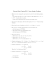### A family of newforms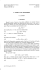### Problem Set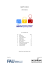### 8.4 Dividing Integers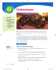### Tauber Arons, Inc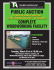### Pre-Algebra With Pizzazz### Chapter 8 - SchoolRack### Student Material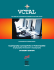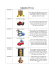### Mathematical Problem Solving for Elementary School Teachers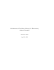### Numeration 2016 - Katedra matematiky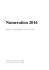### Owner`s Manual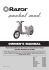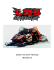### How to Backdoor Diffie-Hellman - Cryptology ePrint Archive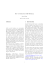### Bridge to Higher Mathematics### Prealgebra 5th Edition - Computer Graphics Home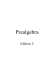### zeitung - Helping a Hero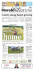### Sarasota, Florida May 2016